시간 제한 메모리 제한 제출 정답 맞은 사람 정답 비율
30 초 (추가 시간 없음) 1024 MB (추가 메모리 없음) 3 3 3 100.000%

## 문제

Emmett found an old clock in his attic. The clock is a circle with 3 hands that attach to the center and rotate clockwise at constant speeds. They are called the hours hand, the minutes hand and the seconds hand. At midnight, all hands point up. The hours hand completes a full revolution in 12 hours, the minutes hand completes a full revolution in 1 hour, and the seconds hand completes a full revolution in 1 minute. 1 hour is equal to 60 minutes, 1 minute is equal to 60 seconds, and 1 second is equal to 109 nanoseconds.

For example, the clock depicted below is showing that the time is exactly 6 hours and 30 minutes after midnight. The hours hand (short black) is halfway between 6 and 7 (completed 6.5/12 of a revolution), the minutes hand (long black) is pointing straight down because it has completed exactly 6 and a half full revolutions and the seconds hand (red) is pointing straight up because it has completed an integer number of full revolutions.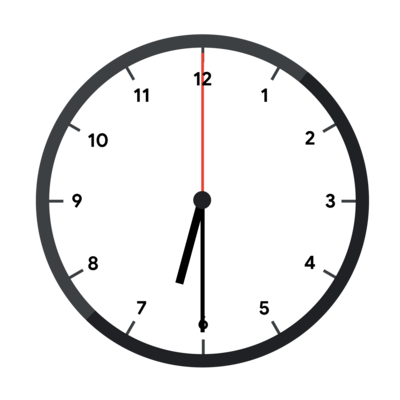Unfortunately, the hands are broken, so they all look identical and there is no way to know which hand is which. The clock in the picture above, with its hands broken, would look like this.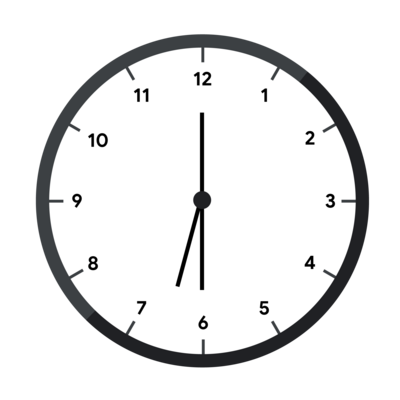In addition, no markings remain that allow Emmett to know which way is up, so any rotation of the clock could be the correct one (the clock can only be rotated, not reflected). To continue with our example, the fully broken clock could look like this.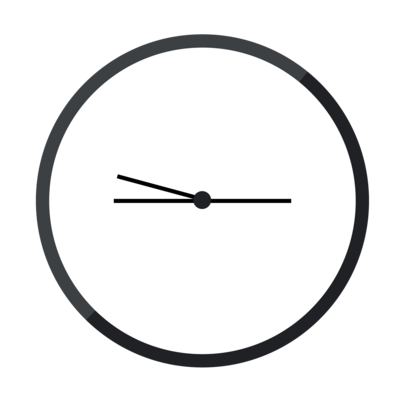Emmett does know that the time was strictly before noon, that is, strictly less than 12 hours after midnight. Emmett has taken a picture of the clock. Given that picture (represented by the angles of the hands relative to a single arbitrary axis), figure out what time it could correspond to.

Notice that Emmett has already figured out a viable orientation of the clock in some cases (Test Set 1) and has managed to narrow down the possible times to a whole integer number of seconds (Test Sets 1 and 2) or nanoseconds (Test Set 3). Please see the limits sections for more details.

## 입력

The first line of the input gives the number of test cases, T. T lines follow. Each line describes a test case and contains three integers A, B, and C: the angles of each hand, relative to an arbitrary axis and given in ticks in the clockwise direction. 1 tick is equal to 1/12×10−10 degrees. This means that the hours hand rotates exactly 1 tick each nanosecond, the minutes hand rotates exactly 12 ticks each nanosecond and the seconds hand rotates exactly 720 ticks each nanosecond.

## 출력

For each test case, output one line containing Case #x: h m s n, where x is the test case number (starting from 1) and h, m, s, and n are integers: h is the number of full hours since midnight (between 0 and 11, inclusive), m is the number of full minutes since the last full hour (between 0 and 59, inclusive), s is the number of full seconds since the last full minute (between 0 and 59, inclusive) and n is the number of full nanoseconds since the last full second (between 0 and 109−1, inclusive).

## 제한

• 1 ≤ T ≤ 100.
• 0 ≤ A ≤ B ≤ C < 360×12×1010.

## Test Set 1 (5점)

There is a time t that corresponds to the input such that:

• t is an integer number of seconds after midnight.
• t can be read from the input clock without rotating it.

## Test Set 2 (6점)

There is a time that corresponds to the input and is an integer number of seconds after midnight.

## Test Set 3 (19점)

There is a time that corresponds to the input and is an integer number of nanoseconds after midnight.

## 예제 입력 1

3
0 0 0
0 21600000000000 23400000000000
1476000000000 2160000000000 3723000000000


## 예제 출력 1

Case #1: 0 0 0 0
Case #2: 6 30 0 0
Case #3: 1 2 3 0


In Sample Case #1, all hands point up (as in the first picture below) which happens only exactly at midnight (as in the second picture below).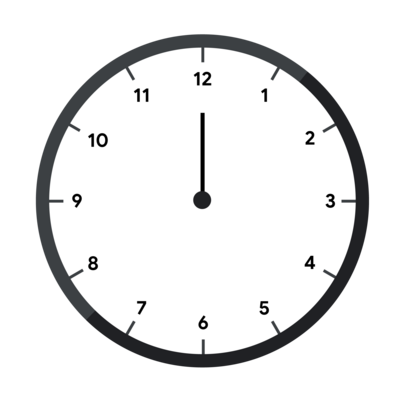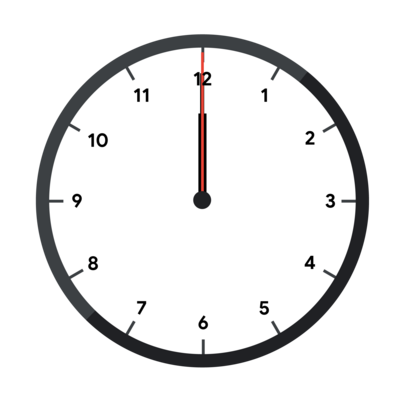Sample Case #2 is the one pictured in the main part of the statement. The angles of the hands in degrees are 0, 180 and 195. These angles can correspond to 6⁠h 30⁠m 0⁠s without rotating the clock, as the pictures in the main part of the statement show. Notice however, that at 0⁠h 30⁠m 0⁠s (pictured below), the clock looks the same but rotated 180 degrees.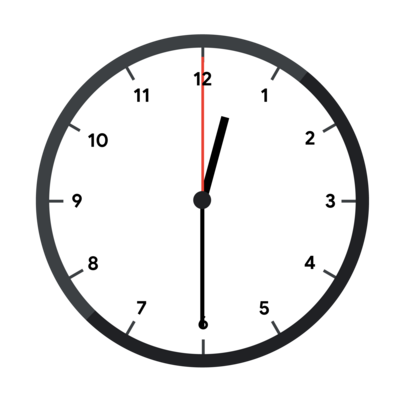Even in Test Set 1, 0⁠h 30⁠m 0⁠s would be a valid answer. The limit only says that there is one valid time that does not require rotating the clock, but times that work with rotation are also valid answers.

In Sample Case #3, the input represents the clock in the first picture below and the given output happens when interpreting the clock as in the second picture below.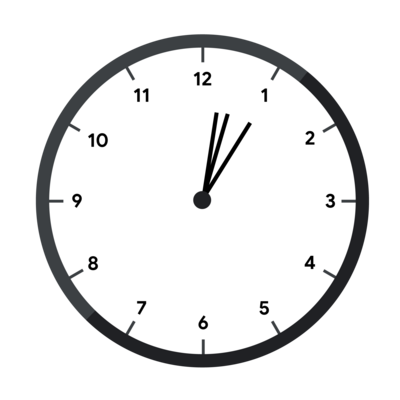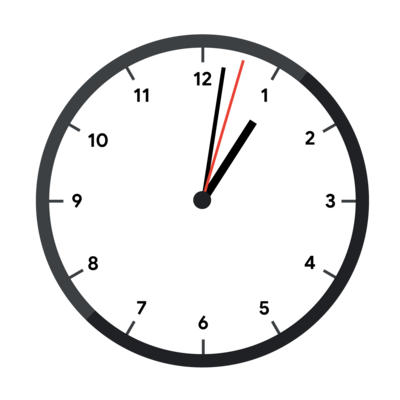## 예제 입력 2

3
5400000000000 5400000000000 5400000000000
10800000000000 32400000000000 34200000000000
23076000000000 23760000000000 25323000000000


## 예제 출력 2

Case #1: 0 0 0 0
Case #2: 0 30 0 0
Case #3: 1 2 3 0


Sample Cases in this test set are the same as in the previous one, but the clock is rotated by 45, 90, and 180 degrees clockwise respectively, as shown below.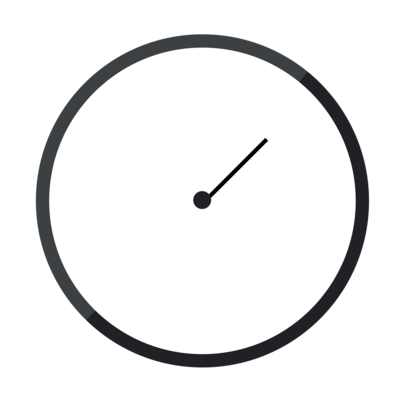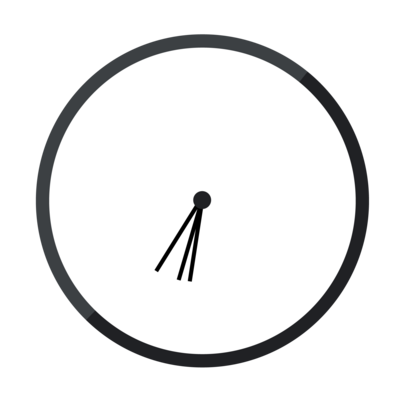## 예제 입력 3

1
0 11 719


## 예제 출력 3

Case #1: 0 0 0 1


As explained above, 1 nanosecond after midnight the hands are moved by 1, 12, and 720 ticks, respectively. If the clock is also rotated counter-clockwise by 1 tick, the hand angles are exactly the ones given in the input.

## 채점 및 기타 정보

• 예제는 채점하지 않는다.